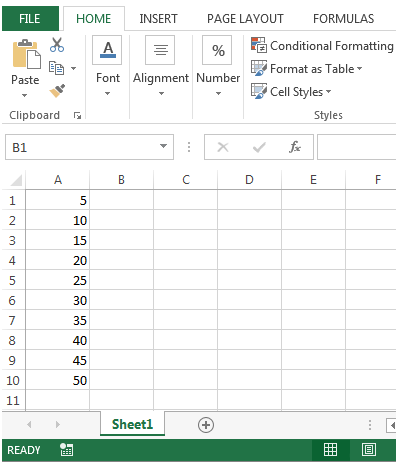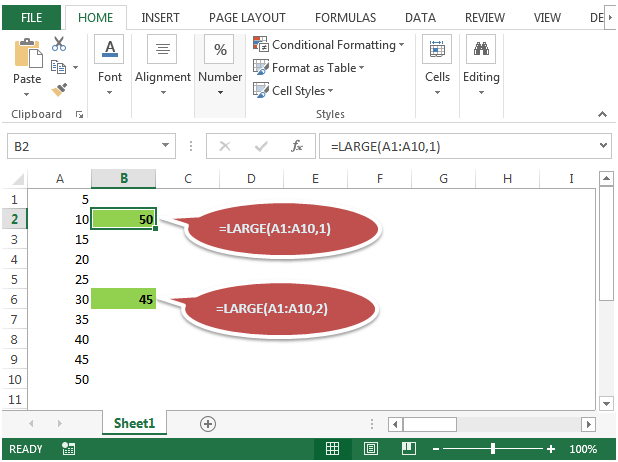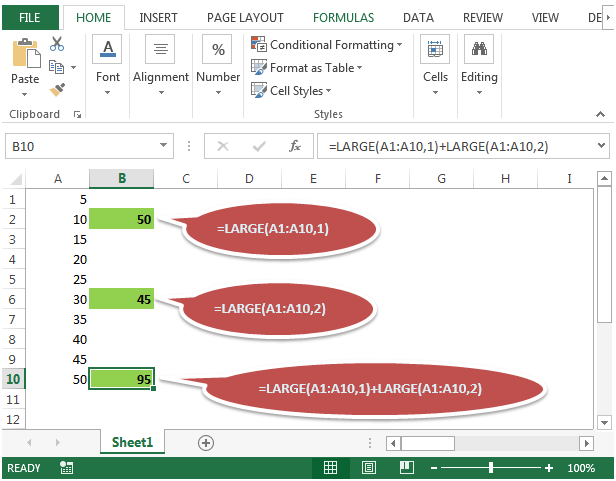# Sum the largest two numbers in range in Microsoft Excel 2010

In this article, we will learn how to sum the largest two numbers in a range in Microsoft Excel 2010.

In order to calculate the sum of two largest numbers in any range, we will use a combination of SUM & LARGE functions.

SUM: Adds all the numbers in a range of cells

Syntax: =SUM(number1,number2,...)

number1: The first argument that you want to add.

number2: The second argument that you want to add.

There can be maximum 255 arguments. Refer below screenshot:LARGE: Returns the k-th largest value in a data set. For example, you can use LARGE function to return the highest marks scored in a class.

Syntax: =LARGE(array,k)

array: The array or range of cells for which you want to find the k-th largest value.

k: Itis the kth position from the largest value to return in the array or range of cells.

To calculate the sum of two largest numbers, we have to take an example:

• We have some numbers in column A• We will first use Large function to find out the highest & second highest value in the given range of cells.
• We will use formula =LARGE(A1:A10,1) to get the highest value &=LARGE(A1:A10,2) and second highest value. The output we get is 50 & 45• Adding both formulas in a single cell we will get the output in B10=LARGE(A1:A10,1)+LARGE(A1:A10,2)• We can merge SUM function with LARGE function to make a formula as=SUM(LARGE(A1:A10,1),LARGE(A1:A10,2))
Or
=SUM(LARGE(A1:A10,{1,2}))

Both are yielding the same result i.e. 95.

1.=SUM(2+4,10,3) WHAT IS THE RESULT IN EXCEL

2.Hi Kiran,

You can’t find the largest number from a 1 digit value. And if you wish to calculate only one largest value it should be a two digit value or two number value in the range, only then you can find the largest value.

Ex:

20, 10 = large(A1:A2,1)

3.Hello,

While adding largest of two number the minimum parameter should be 2 in some cases will not have minimum parameter so what we should do.
Ex:

10 20 3 22 =sum(large(range,1)+large(range,2)) = 42

20 =sum(large(range,1)+large(range,2)) = Num

error?

If it is the case it should calculate only largest of one number and give value as 20, for this what i should do???

Thank You

4.Question. I understand this, and I appreciate it, but how would I go about using these LARGE fomula's to find the largest sum of any two CONSECUTIVE numbers?

5.Terry O'Neill

Fantastic! What a job you have saved me in my Golf Society Summer League - best 5 out of 15 results per competitor!!
Terry

6.1.find sum of 2 larger number out of 20 numbers

=SUM(LARGE(A1:A20,{1,2}))

2.find sum of 3 larger number out of 30 range

=SUM(LARGE(D1:D30,{1,2,3}))

7.where C3 is n

8.=SUMIF(A1:A10,">="&LARGE(A1:A10,C3))

9.could that format be used to set the property to -1 (locked) ?

10."The formula given is incorrect.